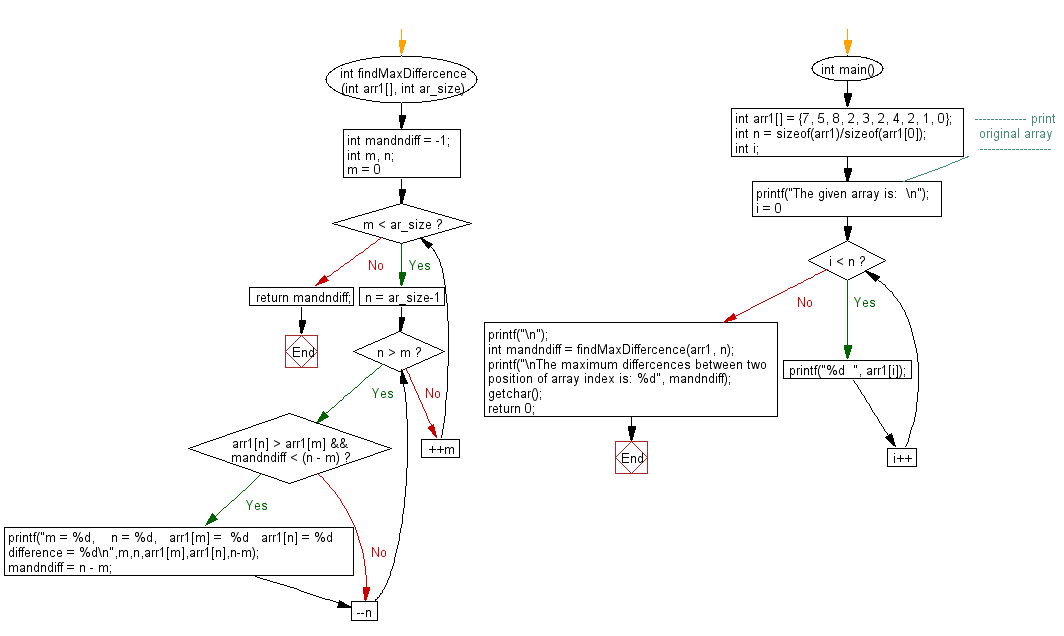﻿ C exercises: Find the maximum n – m such that array[n] > array[m] from a given array[] - w3resource# C Exercises: Find the maximum n – m

## C Array: Exercise-88 with Solution

Write a program in C to find the maximum n – m such that array[n] > array[m] from a given array[]. Given an array arr[], find the maximum j – i such that arr[j] > arr[i].

Sample Solution:

C Code:

``````#include <stdio.h>
int findMaxDiffercence(int arr1[], int ar_size)
{
int mandndiff = -1;
int m, n;

for (m = 0; m < ar_size; ++m)
{
for (n = ar_size-1; n > m; --n)
{
if(arr1[n] > arr1[m] && mandndiff < (n - m))
{
printf("m = %d,    n = %d,   arr1[m] =  %d   arr1[n] = %d    difference = %d\n",m,n,arr1[m],arr1[n],n-m);
mandndiff = n - m;
}
}
}

return mandndiff;
}

int main()
{
int arr1[] = {7, 5, 8, 2, 3, 2, 4, 2, 1, 0};
int n = sizeof(arr1)/sizeof(arr1);
int i;
//------------- print original array ------------------
printf("The given array is:  \n");
for(i = 0; i < n; i++)
{
printf("%d  ", arr1[i]);
}
printf("\n");
//-----------------------------------------------------------
int mandndiff = findMaxDiffercence(arr1, n);
printf("\nThe maximum differcences between two position of array index is: %d", mandndiff);
getchar();
return 0;
}
```
```

Sample Output:

```The given array is:
7  5  8  2  3  2  4  2  1  0
m = 0,    n = 2,   arr1[m] =  7   arr1[n] = 8    difference = 2
m = 3,    n = 6,   arr1[m] =  2   arr1[n] = 4    difference = 3

The maximum differcences between two position of array index is: 3
```

Flowchart:C Programming Code Editor:

Improve this sample solution and post your code through Disqus.

﻿

## C Programming: Tips of the Day

Where is the C auto keyword used?

auto is a modifier like static. It defines the storage class of a variable. However, since the default for local variables is auto, you don't normally need to manually specify it.

Ref : https://bit.ly/3yzwC9r